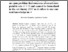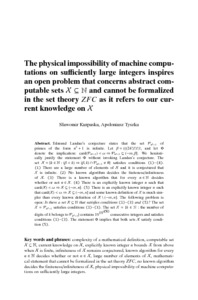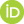# The physical impossibility of machine computations on sufficiently large integers inspires an open problem that concerns abstract computable sets X⊆N and cannot be formalized in the set theory ZFC as it refers to our current knowledge on X

Kurpaska, Sławomir and Tyszka, Apoloniusz (2020) The physical impossibility of machine computations on sufficiently large integers inspires an open problem that concerns abstract computable sets X⊆N and cannot be formalized in the set theory ZFC as it refers to our current knowledge on X. [Preprint]Preview
Text
machine_computations.pdf

## Abstract

Edmund Landau's conjecture states that the set P(n^2+1) of primes of the form n^2+1 is infinite. Let β=(((24!)!)!)!, and let Φ denote the implication: card(P(n^2+1))<ω ⇒ P(n^2+1)⊆(-∞,β]. We heuristically justify the statement Φ without invoking Landau's conjecture. The set X = {k∈N: (β<k) ⇒ (β,k)∩P(n^2+1)≠∅} satisfies conditions (1)-(4). (1) There are a large number of elements of X and it is conjectured that X is infinite. (2) No known algorithm decides the finiteness/infiniteness of X . (3) There is a known algorithm that for every n∈N decides whether or not n∈X. (4) There is an explicitly known integer n such that card(X)<ω ⇒ X⊆(-∞,n]. (5) There is an explicitly known integer n such that card(X)<ω ⇒ X⊆(-∞,n] and some known definition of X is much simpler than every known definition of X\(-∞,n]. The following problem is open: Is there a set X⊆N that satisfies conditions (1)-(3) and (5)? The set X=P(n^2+1) satisfies conditions (1)-(3). The set X={k∈N: the number of digits of k belongs to P(n^2+1)} contains 10^{10^{450}} consecutive integers and satisfies conditions (1)-(3). The statement Φ implies that both sets X satisfy condition (5).

 Export/Citation: EndNote | BibTeX | Dublin Core | ASCII/Text Citation (Chicago) | HTML Citation | OpenURL
 Social Networking:

Item Type: Preprint
Creators:
CreatorsEmailORCIDTyszka, Apoloniuszrttyszka@cyf-kr.edu.pl0000-0002-2770-5495
Keywords: complexity of a mathematical definition; computable set X⊆N; current knowledge on X; explicitly known integer n bounds X from above when X is finite; infiniteness of X remains conjectured; known algorithm for every n∈N decides whether or not n∈X; large number of elements of X; mathematical statement that cannot be formalized in the set theory ZFC; no known algorithm decides the finiteness/infiniteness of X; physical impossibility of machine computations on sufficiently large integers
Subjects: Specific Sciences > Mathematics > Foundations
Specific Sciences > Mathematics > Logic
Depositing User: Apoloniusz Tyszka
Date Deposited: 20 Jun 2020 18:19
Item ID: 17352
Subjects: Specific Sciences > Mathematics > Foundations
Specific Sciences > Mathematics > Logic
Date: 21 April 2020
URI: http://philsci-archive.pitt.edu/id/eprint/17352

### Monthly Views for the past 3 yearsView Item